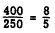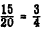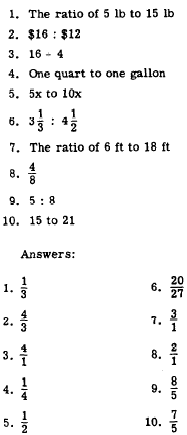Ratio, Proportion, and VariationCustom SearchCHAPTER 13 RATIO, PROPORTION, AND VARIATION The solution of problems based on ratio, proportion, and variation involves no new principles. However, familiarity with these topics will often lead to quick and simple solutions to problems that would otherwise be more complicated. RATIO The results of observation or measurement often must be compared with some standard value in order to have any meaning. For example, to say that a man can read 400 words per minute has little meaning as it stands. However, when his rate is compared to the 250 words per minute of the average reader, one can see that he reads considerably faster than the average reader. How much faster? To find out, his rate is divided by the average rate, as follows:Thus, for every 5 words read by the average reader, this man reads 8. Another way of making this comparison is to say that he reads 1 3/5 times as fast as the average reader. When the relationship between two numbers is shown fn this way, they are compared as a RATIO. A ratio is a comparison of two like quantities. It is the quotient obtained by dividing the first number of a comparison by the second. Comparisons may be stated in more than one way. For example, if one gear has 40 teeth and another has 10, one way of stating the comparison would be 40 teeth to 10 teeth. This comparison could be shown as a ratio in four ways as follows:When the emphasis is on "ratio," all of these expressions would be read, "the ratio of 40 to 10." The form 40 � 10 may also be read "40 divided by 10." The form g may also be read, "40 over 10." Comparison by means of a ratio is limited to quantities of the same kind. For example, in order to express the ratio between 6ft and 3yd, both quantities must be written in terms of the same unit. Thus the proper form of this ratio is 2 yd : 3 yd, not 6 ft : 3 yd. When the parts of the ratio are expressed in terms of the same unit, the units cancel each other and the ratio consists simply of two numbers. In this example, the final form of the ratio is 2 : 3. Since a ratio is also a fraction, all the rules that govern fractions may be used in working with ratios. Thus, the terms may be reduced, increased, simplified, and so forth, according to the rules for fractions. To reduce the ratio 15:20 to lowest terms, write the ratio as a fraction and then proceed as for fractions. Thus, 15:20 becomesHence the ratio of 15 to 20 is the same as the ratio of 3 to 4.  Notice the distinction in thought between 3/4 as a fraction and 3/4 as a ratio, As a fraction we think of 3/4 as the single quantity "three-fourths." As a ratio, we think of 3/4 as a comparison between the two numbers, 3 and 4. For example, the lengths of two sides of a triangle are 1 9/16 ft and 2 ft. To compare these lengths by means of a ratio, divide one number by the other and reduce to lowest terms, as follows:The two sides of the triangle compare as 25 to 32. INVERSE RATIO It is often desirable to compare the numbers of a ratio in the inverse order. To do this, we simply interchange the numerator and the de- nominator. Thus, the inverse of 15:20 is 20:15. When the terms of a ratio are interchanged, the INVERSE RATIO results. Practice problems, In problems 1 through 6, write the ratio as a fraction and reduce to lowest terms. In problems 7 through 10, write the inverse of the given ratio.Integrated Publishing, Inc. - A (SDVOSB) Service Disabled Veteran Owned Small Business